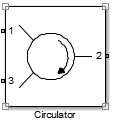Main Content

# Circulator

Model ideal frequency-independent circulators with S-parameters

• Library:
• RF Blockset / Circuit Envelope / Junctions

•## Description

Use the Circulator block to model ideal passive three-port circulators in a circuit envelope environment. Circulators are used to control signal direction and flow inside an RF circuit. The Circulator block can circulate the signal in clockwise or counterclockwise direction depending on the parameter selection.

## Parameters

expand all

Circulator direction, specified as:

• `Circulator clockwise`

The default option is `Circulator clockwise`. The s-parameter matrix for `Circulator clockwise` is:

$\left[\begin{array}{ccc}0& 0& 1\\ 1& 0& 0\\ 0& 1& 0\end{array}\right]$

`Circulator counter clockwise`

The s-parameter matrix for `Circulator counter clockwise` is:

$\left[\begin{array}{ccc}0& 1& 0\\ 0& 0& 1\\ 1& 0& 0\end{array}\right]$

• `Tee H-plane (S11=0)`

The s-parameter matrix for `Tee H-plane (S11=0)` is:

$\left(\begin{array}{ccc}0& 1& 0\\ 1& 0& 0\\ 0& 0& 1\end{array}\right)$

• `Reciprocal phase shifter`

The s-parameter matrix for ```Reciprocal phase shifter``` is:

$\left(\begin{array}{ccc}0& phas{e}_{12}& 0\\ phas{e}_{12}& 0& 0\\ 0& 0& phas{e}_{33}\end{array}\right)\left(\frac{-j}{\sqrt{2}}\right)$

Reference impedance of circulator, specified as a scalar or three-tuple vector.

Angle to shift the phase of the signal in port 1 and port 2, specified as a positive scalar in radians.

Angle to shift the phase of the signal in port 3, specified as a positive scalar in radians.

Select this parameter to ground and hide the negative terminals. To expose the negative terminals, clear this parameter. By exposing these terminals, you can connect them to other parts of your model.

By default, this option is selected.

## See Also

Introduced in R2014a

## Support

#### Model RF Power Amplifiers and Increase Transmitter Linearity with DPD Using MATLAB

Download white paper